# mlr (`pip install mlr`)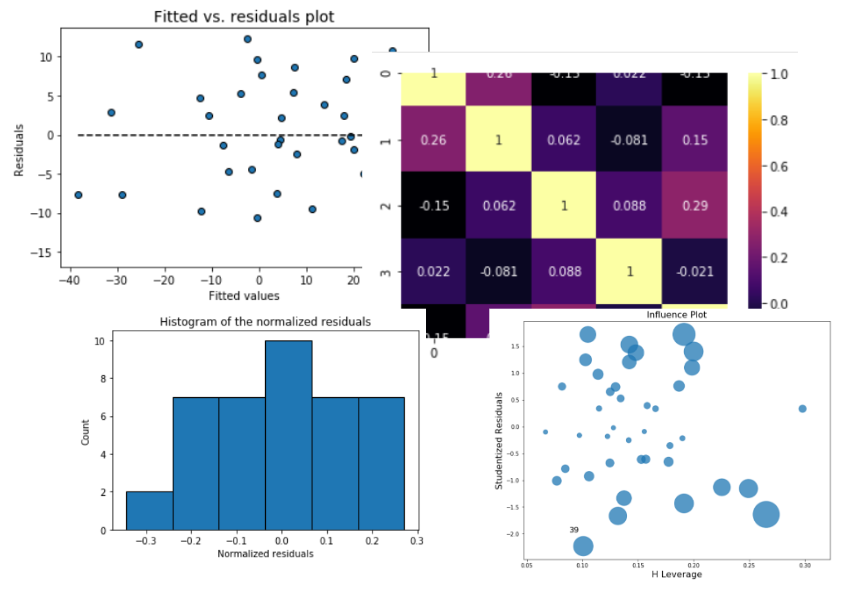A lightweight, easy-to-use Python package that combines the `scikit-learn`-like simple API with the power of statistical inference tests, visual residual analysis, outlier visualization, multicollinearity test, found in packages like `statsmodels` and R language.

Authored and maintained by Dr. Tirthajyoti Sarkar (Website, LinkedIn profile)

### Useful regression metrics,

• MSE, SSE, SST
• AIC (Akaike Information Criterion), and BIC (Bayesian Information Criterion)

### Inferential statistics,

• Standard errors
• Confidence intervals
• p-values
• t-test values
• F-statistic

### Visual residual analysis,

• Plots of fitted vs. features,
• Plot of fitted vs. residuals,
• Histogram of standardized residuals
• Q-Q plot of standardized residuals

### Outlier detection

• Influence plot
• Cook's distance plot

### Multicollinearity

• Pairplot
• Variance infletion factors (VIF)
• Covariance matrix
• Correlation matrix
• Correlation matrix heatmap

## Requirements

• numpy (`pip install numpy`)
• pandas (`pip install pandas`)
• matplotlib (`pip install matplotlib`)
• seaborn (`pip install seaborn`)
• scipy (`pip install scipy`)
• statsmodels (`pip install statsmodels`)

## Install

(On Linux and Windows) You can use `pip`

`pip install mlr`

(On Mac OS), first install pip,

``````curl https://bootstrap.pypa.io/get-pip.py -o get-pip.py
python get-pip.py
``````

Then proceed as above.

For the latest additions, you can always clone this Github repo and run the setup.py.

## Quick Start

Import the `MyLinearRegression` class,

``````from mlr.MLR import MyLinearRegression as mlr
import numpy as np
``````

Generate some random data

``````num_samples=40
num_dim = 5
X = 10*np.random.random(size=(num_samples,num_dim))
coeff = np.array([2,-3.5,1.2,4.1,-2.5])
y = np.dot(coeff,X.T)+10*np.random.randn(num_samples)
``````

Make a model instance,

``````model = mlr()
``````

Ingest the data

``````model.ingest_data(X,y)
``````

Fit,

``````model.fit()
``````

## Directly read from a Pandas DataFrame

You can read directly from a Pandas DataFrame. Just give the features/predictors' column names as a list and the target column name as a string to the `fit_dataframe` method.

At this point, only numerical features/targets are supported but in future releases we will support categorical variables too.

``````<... obtain a Pandas DataFrame by some processing>
df = pd.DataFrame(...)
feature_cols = ['X1','X2','X3']
target_col = 'output'

model = mlr()
model.fit_dataframe(X=feature_cols,y = target_col,dataframe=df)
``````

## Metrics

So far, it looks similar to the linear regression estimator of Scikit-Learn, doesn't it?
Here comes the difference,

### Print all kinds of regression model metrics, one by one,

``````print ("R-squared: ",model.r_squared())
print("MSE: ",model.mse())

>> R-squared:  0.8344327025902752
MSE:  72.2107655649954

``````

### Or, print all the metrics at once!

``````model.print_metrics()

>> sse:     2888.4306
sst:     17445.6591
mse:     72.2108
r^2:     0.8344
AIC:     296.6986
BIC:     306.8319
``````

## Correlation matrix, heatmap, covariance

We can build the correlation matrix right after ingesting the data. This matrix gives us an indication how much multicollinearity is present among the features/predictors.

### Correlation matrix

``````model.ingest_data(X,y)
model.corrcoef()

>> array([[ 1.        ,  0.18424447, -0.00207883,  0.144186  ,  0.08678109],
[ 0.18424447,  1.        , -0.08098705, -0.05782733,  0.19119872],
[-0.00207883, -0.08098705,  1.        ,  0.03602977, -0.17560097],
[ 0.144186  , -0.05782733,  0.03602977,  1.        ,  0.05216212],
[ 0.08678109,  0.19119872, -0.17560097,  0.05216212,  1.        ]])
``````

### Covariance

``````model.covar()

>> array([[10.28752086,  1.51237819, -0.01770701,  1.47414685,  0.79121778],
[ 1.51237819,  6.54969628, -0.5504233 , -0.47174359,  1.39094876],
[-0.01770701, -0.5504233 ,  7.05247111,  0.30499622, -1.32560195],
[ 1.47414685, -0.47174359,  0.30499622, 10.16072256,  0.47264283],
[ 0.79121778,  1.39094876, -1.32560195,  0.47264283,  8.08036806]])
``````

### Correlation heatmap

``````model.corrplot(cmap='inferno',annot=True)
``````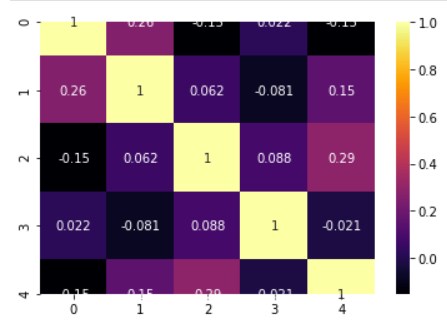## Statistical inference

### Perform the F-test of overall significance

It retunrs the F-statistic and the p-value of the test.

If the p-value is a small number you can reject the Null hypothesis that all the regression coefficient is zero. That means a small p-value (generally < 0.01) indicates that the overall regression is statistically significant.

``````model.ftest()

>> (34.270912591948814, 2.3986657277649282e-12)
``````

### How about p-values, t-test statistics, and standard errors of the coefficients?

Standard errors and corresponding t-tests give us the p-values for each regression coefficient, which tells us whether that particular coefficient is statistically significant or not (based on the given data).

``````print("P-values:",model.pvalues())
print("t-test values:",model.tvalues())
print("Standard errors:",model.std_err())

>> P-values: [8.33674608e-01 3.27039586e-03 3.80572234e-05 2.59322037e-01 9.95094748e-11 2.82226752e-06]
t-test values: [ 0.21161008  3.1641696  -4.73263963  1.14716519  9.18010412 -5.60342256]
Standard errors: [5.69360847 0.47462621 0.59980706 0.56580141 0.47081187 0.5381103 ]

``````

### Confidence intervals

``````model.conf_int()

>> array([[-10.36597959,  12.77562953],
[  0.53724132,   2.46635435],
[ -4.05762528,  -1.61971606],
[ -0.50077913,   1.79891449],
[  3.36529718,   5.27890687],
[ -4.10883113,  -1.92168771]])

``````

## Visual analysis of the residuals

Residual analysis is crucial to check the assumptions of a linear regression model. `mlr` helps you check those assumption easily by providing straight-forward visual analytis methods for the residuals.

### Fitted vs. residuals plot

Check the assumption of constant variance and uncorrelated features (independence) with this plot

``````model.fitted_vs_residual()
``````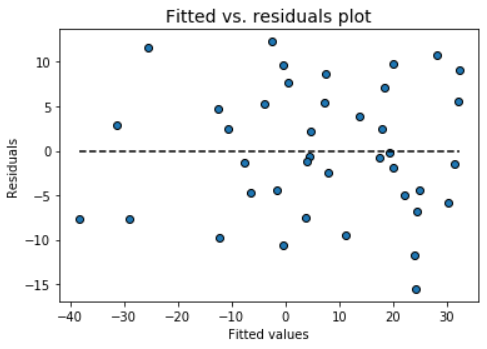### Fitted vs features plot

Check the assumption of linearity with this plot

``````model.fitted_vs_features()
``````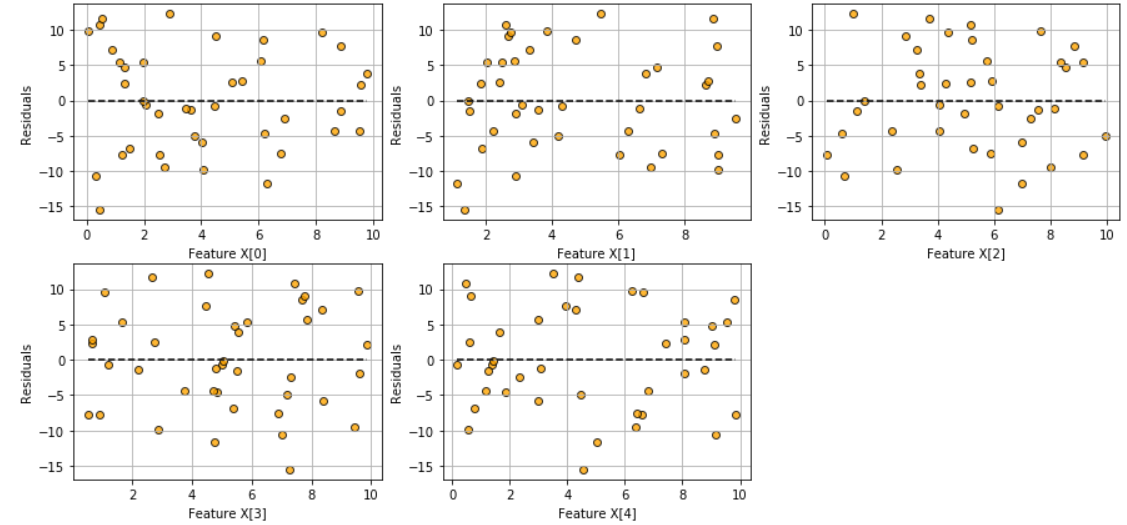### Histogram and Q-Q plot of standardized residuals

Check the normality assumption of the error terms using these plots,

``````model.histogram_resid()
``````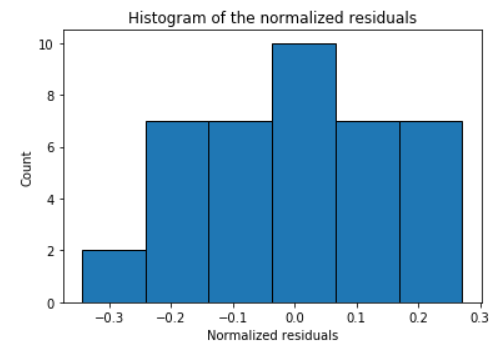``````model.qqplot_resid()
``````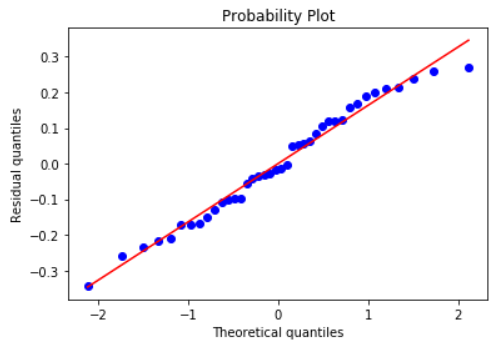## Do more

Do more fun stuff with your regression model. More features will be added in the future releases!

• Outlier detection and plots
• Multicollinearity checks

Get A Weekly Email With Trending Projects For These Topics
No Spam. Unsubscribe easily at any time.
Python (1,137,610
Machine Learning (30,619
Data Science (8,743
Statistics (4,280
Analytics (2,176
Scikit Learn (1,947
Regression (1,308
Linear Regression (1,190
Modeling (677
Data Analytics (407
Statistical Analysis (336
Predictive Modeling (334
Related Projects